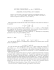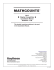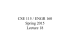# Discrete Math, Spring 2013 - Some Sample Problems

## Transcription

Discrete Math, Spring 2013 - Some Sample Problems
```Discrete Math, Spring 2013 - Some Sample Problems
1. Write down the contrapositive and converse of each of the the following implications.
a. If a triangle with sides a, b, c (with c largest) is right-angled then a2 + b2 = c2 .
b. If 2n − 1 is prime then n is prime.
c. If the wind is blowing from the south then the humidity is low.
2. Let b and c be odd integers. Show that the equation x2 + bx + c = 0 has no integer
solutions.
3. Prove that for any real numbers x and y if x + y ≥ 2 then x ≥ 1 or y ≥ 1.
4. For any set S, recall that P(S) denotes the set of all subsets of S.
a. List the elements of P(P({∅})).
b. How many elements does P(P(P({∅}))) have?
c. List the elements of P(P(P({∅}))).
5. Let S and T be sets.
a. Show that (S \ T ) ∪ (T \ S) = (S ∪ T ) \ (S ∩ T ).
b. Show that (S \ T ) ∩ (T \ S) = ∅.
c. Show that S ∪ T = S ∩ T if and only if S = T .
6. Consider the functions f : R → R and g : R → R given by f (x) = αx + β and
g(x) = 2x − 3. Determine the numbers α and β if f ◦ g = g ◦ f .
7. Consider the function f : R → R given by
(
x,
if x ∈ Q,
f (x) =
x + 1, if x ∈
/ Q.
Show that f is a bijection and find f −1 .
8. Consider the function g : R → R given by
(
x2 ,
if x ∈ Q,
f (x) =
x + 1, if x ∈
/ Q.
a. Is f injective?
b. Is f surjective?
2
9. a. Prove that the set of all polynomials with integer coefficients is countable.
b. Show that the set of all irrational real numbers is uncountable.
10. Let a and b be any real numbers with a < b. Show that there is a bijection from
the interval (0, 1) to the interval (a, b).
11. Show that {(x, y) ∈ R2 : x2 + y 2 = 1} (the unit circle) is uncountable.
12. a. Prove that 2n + 1 ≤ 2n for all integers n ≥ 3.
b. Prove that nn+1 > (n + 1)n for all integers n ≥ 3.
Pn
c. Prove that k=1 k k! = (n + 1)! − 1 for all integers n ≥ 1.
13. Find the greatest common divisor d of 552 and 713 and find integers x and y such
that d = 552x + 713y.
14. Determine 3301 (mod 11) and 5110 (mod 13).
15. Let p be a prime number.
a. Show that if x2 ≡ x (mod p) then x ≡ 0 (mod p) or x ≡ 1 (mod p).
b. Suppose an integer a is not divisible by p. Show that ax ≡ 1 (mod p) has a
solution x in Z.
16. Let a, b, c be positive integers such that a | c and b | c.
a. Show that if (a, b) = 1 then ab | c.
b. Given an example to that ab need not divide c if a and b are not relatively
prime.
17. Show that n7 ≡ n (mod 21) for any integer n. [Hint: use Fermat’s little theorem
and part a of the preceding problem.]
18. Let p and q be distinct primes. Show that pq−1 + q p−1 ≡ 1 (mod pq).
19. a. Prove that
√
p is irrational for any prime number p.
b. Prove that log2 3 is irrational.
20. Show that
(n + m)!
is an integer for any non-negative integers n and m.
n! m!
21. a. How many injective functions are there from {1, 2, 3} to {1, 2, 3, 4}?
3
b. How many surjective functions are there from {1, 2, 3, 4} to {1, 2, 3}?
22. The digits 1, 2, 3, 4, 5, 6 are written down in some order to form a six-digit number.
a. How many such numbers are there in all?
b. How many such numbers are even?
c. How many such numbers are divisible by 5?
d. How many such numbers are divisible by 4?
23. What is the coefficient of x15 in (1 + x)18 ?
n
n
24. a. Explain why
=
(for any nonnegative integers n and k with
k
n−k
k ≤ n).
b. Prove the identity
n 2
X
n
k=0
n
k
=
2n
n
by comparing coefficents of x in (1 + x)n (1 + x)n = (1 + x)2n .
c. Give a combinatorial proof of the identity in part b. [Hint: split a pile of 2n
objects into two piles of equal size and note that to choose n objects from the
piles, you must choose k objects from one pile and n − k from the other.]
```

#### Similar documents### armedforces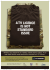### SFWR ENG 2DM3 Discrete Mathematics and Logic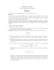### Assignment 2 - Technische Universiteit Eindhoven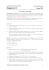### Sample Problems Algebra### Homework 3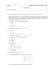### Sample Final Exam Questions (Mandatory Part)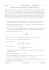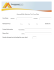### PROBLEM SET 01 - Proofs and induction 1 Proofs 2 Induction### Homework solutions - California State University, Los Angeles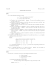### Sample Individual Questions 2012 Canadian Team Mathematics Contest (CTMC)### 2013 School Competition Countdown Round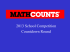### 17) Summa Brasiliensis Mathematicae, II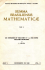### Assignment #3: Boolean Logic / Proof Techniques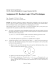### Div2 Problems (Eng)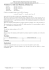### Official solutions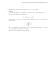### Exercises 09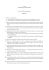### ax + by ≡ 1 MODULO xy ON THE CONGRUENCE